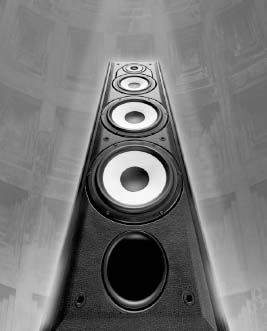NextPrevious

# What are the connections between logarithms and algebra?

Logarithms are the numbers of the power to which a base must be raised in order to get a given positive number. For example, the logarithm of 100 to the base 10 is 2, or log10100 = 2; this is because 102 = 100. Common logarithms are positive numbers that use the number 10 as the base; they are written as log x. Those using the number symbolized by e as the base are called natural logarithms (also phrased as logarithms with a base e); the natural logarithm of a number x is written as ln x.

What’s the connection? Because logarithms are really exponents, they satisfy all the usual rules of exponents. Consequently, tedious and long algebraic calculations such as those involving multiplication and division can be replaced by the simpler processes of adding or subtracting the corresponding logarithms. In general, logarithmic tables are usually used for this purpose—although calculators, computers, and Internet mathematics and computational sites usually replace the need for such tables.Even the simple act of cranking up your sound system involves math. The decibel scale used by your loudspeakers and amplifiers employs the concept of logarithms.

Close

This is a web preview of the "The Handy Math Answer Book" app. Many features only work on your mobile device. If you like what you see, we hope you will consider buying. Get the App